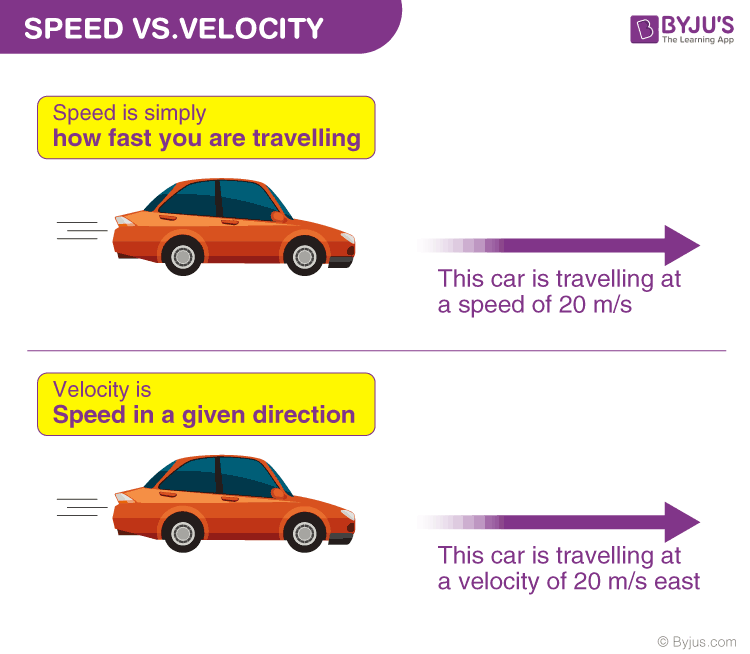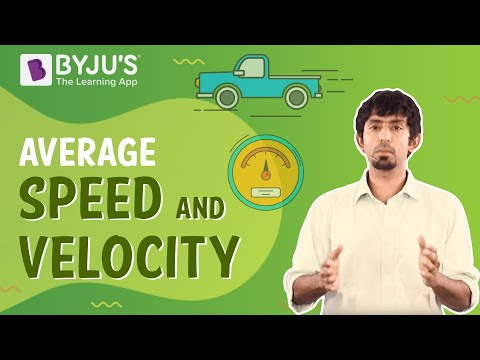# Average Speed and Average Velocity

Before learning about average speed and average velocity, we must know the difference between distance and displacement.

Distance is a scalar quantity which generally implies how much ground has been covered by the object. On the other hand, displacement is a vector quantity, and it is the shortest possible distance between the start and end point.

Example: If a particle is moving in a circle, after one revolution, the distance will be the perimeter of the circle while the displacement would be zero.

Now, let us see what speed and velocity actually are.Speed: Speed is a scalar quantity which means it has no direction. It denotes how fast an object is moving. If the speed of the particle is high it means the particle is moving fast and if it is low,  it means the particle is moving slow.

Velocity: Velocity is a vector quantity which means it has both magnitude and direction. It denotes the rate at which the object is moving or changing position. The direction of the velocity vector is easy to find. Its direction is the same as the direction of the moving object. Even if the object is slowing down and the magnitude of velocity is decreasing, its direction would still be the same as the direction in which the object is moving

## The below video provides the Top 10 NTSE Important Questions on Motion Class 9## Average Speed

The average speed of a body in a certain time interval is the distance covered by the body in that time interval divided by time. So if a particle covers a certain distance s in a time t1 to t2, then the average speed of the body is:

$$\begin{array}{l}v_{av} = \frac{s}{t_2 – t_1}\end{array}$$

In general, average speed formula is:

$$\begin{array}{l}\textup{Average Speed} = \frac{\textup{Total Distance}}{\textup{Total Time}}\end{array}$$

Now, let us look at some of the examples to understand this concept easily

1.) In travelling from Pune to Nagpur, Rahul drove his bike for 2 hours at 60 kmph and 3 hours at 70 kmph.

Sol 1) We know that, Distance = Speed × Time

So, in 2 hours, distance covered = 2 × 60 = 120 km

in the next 3 hours, distance covered = 3 × 70 = 210 km

Total distance covered = 120 + 210 = 330 km

Total time = 2 + 3 = 5 hrs

$$\begin{array}{l}\textup{Average Speed} = \frac{\textup{Total Distance Covered}}{\textup{Time Taken}}\end{array}$$

## Average Velocity

The average velocity of a body in a certain time interval is given as the displacement of the body in that time interval divided by time. So if a particle covers a certain displacement vector AB in a time t1 to t2, then the average velocity of the particle is:

$$\begin{array}{l}\overrightarrow{v_{av}} = \frac{\overrightarrow{AB}}{t_2 – t_1}\end{array}$$
$$\begin{array}{l}\textup{Average Speed} = \frac{\textup{Total Distance}}{\textup{Total Time}}\end{array}$$

Understand the concept of average velocity through the examples given below.

1) What is the average velocity of a person who moves 7 m in 4 seconds and 18 m in 6 seconds along x-axis?

Sol) Initial distance traveled by the person, xi = 7 m,

Final distance traveled, xf  = 18 m,

Initial time interval ti = 4 sec,

Final time interval t= 6 sec,

Average velocity Vav

$$\begin{array}{l}\frac{x_{i}-x_{f}}{t_{f}-t_{i}}= \frac{18-7}{6-4}= \frac{11}{2}\end{array}$$
= 5.5 m/sec.

## The video explains the concepts of average speed and velocity## Frequently Asked Questions – FAQs

Q1

### Speed is a scalar or vector quantity?

Speed is a scalar quantity since it does not specify the direction.
Q2

### What is the formula to calculate the average speed?

Average speed is given by the formula:
Average speed = Total distance / Total time

Q3

### Define velocity.

Velocity is defined as the rate at which the object is moving or changing position.

Q4

### What is the formula to average velocity?

Average velocity = Total displacement / Total time
Q5

### If a particle is moving in a circle, after one revolution, the distance will be the perimeter of the circle. What would be the displacement of the particle?

The displacement would be zero.

## The below video provides the complete quiz of the chapter Motion Class 9